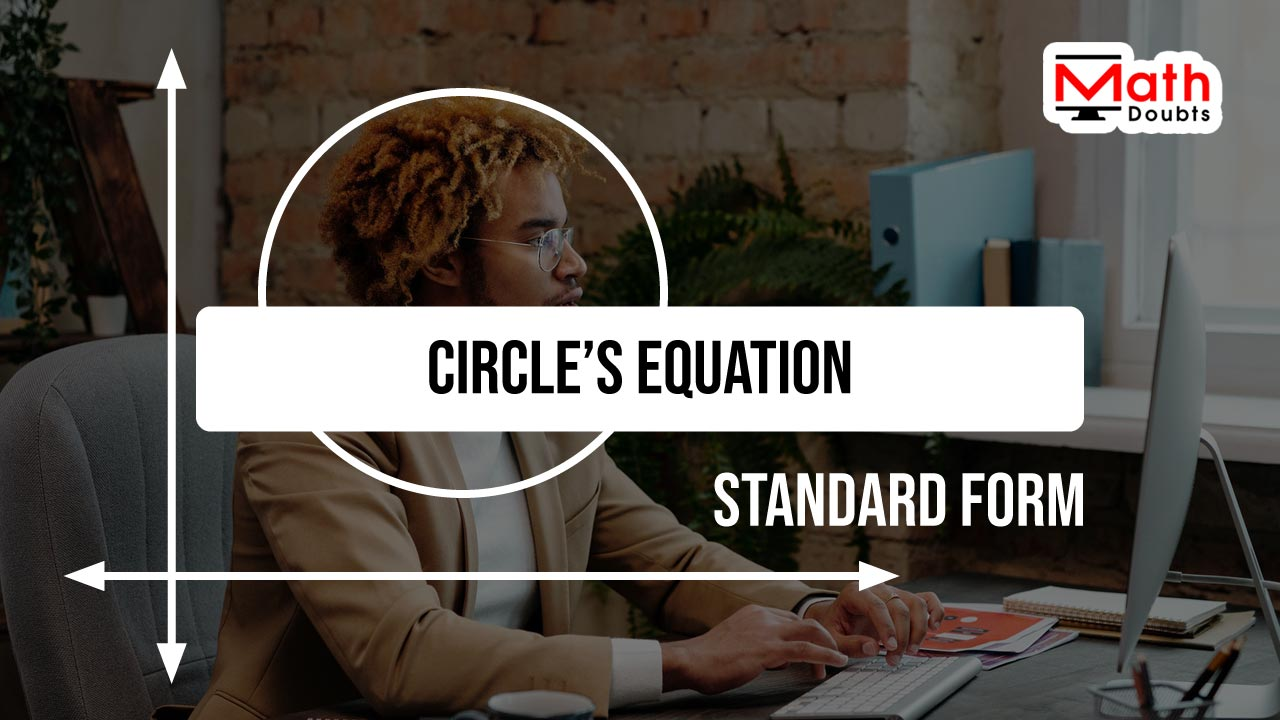# Standard equation of a circle

## Equation

$(x-a)^2+(y-b)^2 \,=\, r^2$

### Introduction

A circle without touching any axis of the two dimensional cartesian coordinate system is a standard form of a circle and its equation is called the equation of a circle in standard form.Let $C$ be a point, which represents the center (or centre) of a circle, $P$ be a point on the circle and the radius of the circle is $r$ units.

Let $a$ and $b$ be the $x$ and $y$ coordinates of center and the centre in coordinate form is written as $C(a, b)$. Similarly, $x$ and $y$ be the coordinates of the point $P$. So, the point $P$ in coordinate form is written as $P(x, y)$ mathematically.

The equation of a circle in standard form is written in mathematics as follows.

#### Simple form

$(x-a)^2+(y-b)^2 \,=\, r^2$

#### Expansion

$x^2+y^2\,-\,2ax\,\,–\,2by+a^2+b^2-r^2 \,=\, 0$

### Other form

The standard equation of a circle is also popularly written in other form by denoting the coordinates of the center with $h$ and $k$. It means, the centre of a circle in coordinate form is $C(h, k)$.

#### Simple form

$(x-h)^2+(y-k)^2 \,=\, r^2$

#### Expansion

$x^2+y^2\,-\,2hx\,\,–\,2ky+h^2+k^2-r^2 \,=\, 0$

##### Proof

Learn how to derive the equation of a circle in general form when the circle does not touch both axes.

Latest Math Topics
Jun 26, 2023
Jun 23, 2023

###### Math Questions

The math problems with solutions to learn how to solve a problem.

Learn solutions

Practice now

###### Math Videos

The math videos tutorials with visual graphics to learn every concept.

Watch now

###### Subscribe us

Get the latest math updates from the Math Doubts by subscribing us.